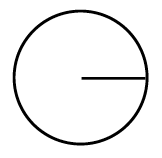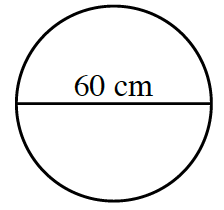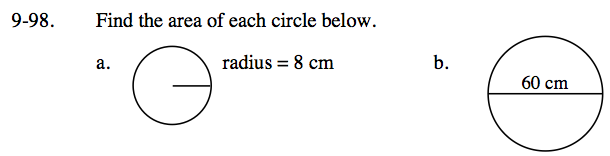Home > CC3 > Chapter 9 > Lesson 9.2.3 > Problem9-98

9-98.
1. Find the area of each circle below. Homework Help ✎

1.radius = 8 cm

2.The formula to find the area of a circle is r2π.

Remember to use the radius and not the diameter.

π · (30 cm)2 = 900π = 2827.43 cm2